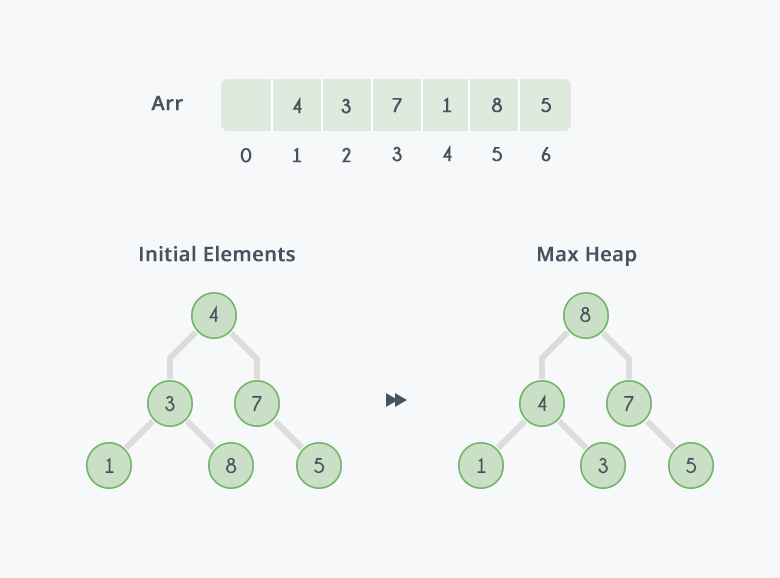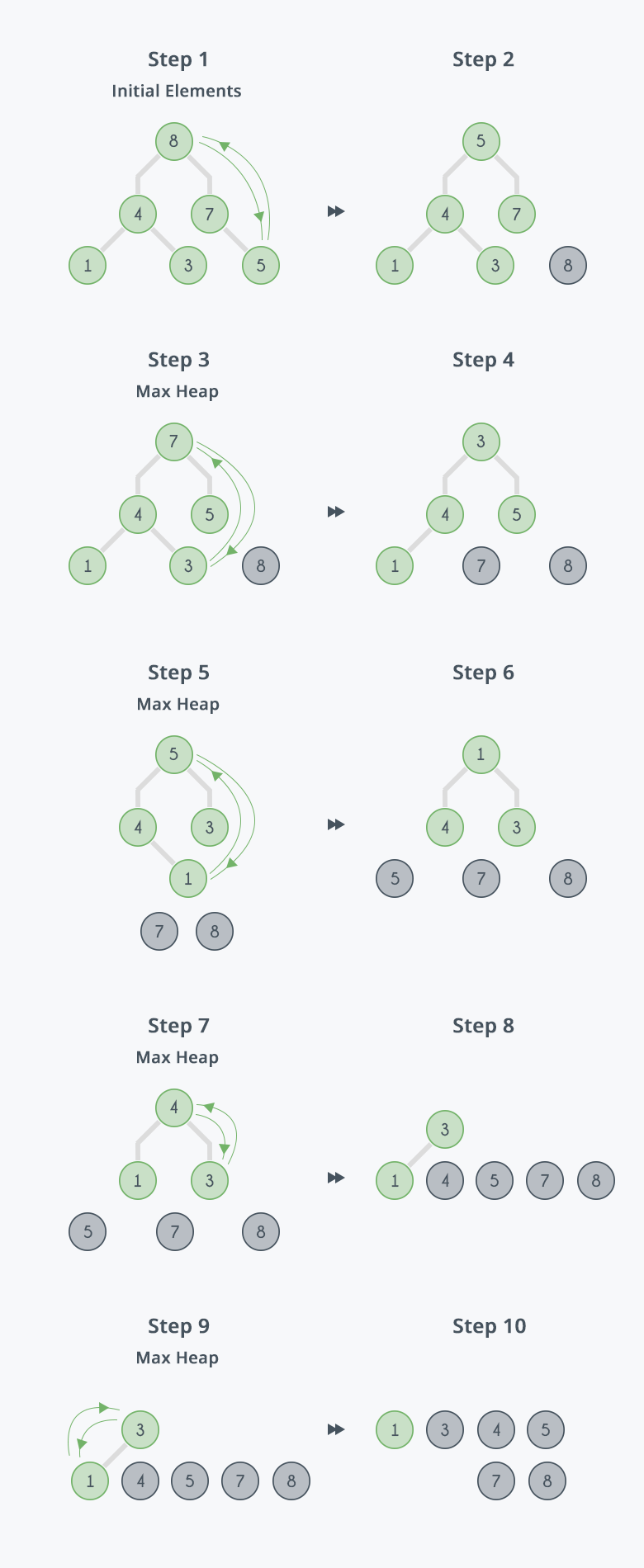Algorithms
Topics:
Heap Sort
• Searching
• Sorting
• Greedy Algorithms
• Graphs
• String Algorithms
• Dynamic Programming

# Heap Sort

Heaps can be used in sorting an array. In max-heaps, maximum element will always be at the root. Heap Sort uses this property of heap to sort the array.

Consider an array $Arr$ which is to be sorted using Heap Sort.

• Initially build a max heap of elements in $Arr$.
• The root element, that is $Arr$, will contain maximum element of $Arr$. After that, swap this element with the last element of $Arr$ and heapify the max heap excluding the last element which is already in its correct position and then decrease the length of heap by one.
• Repeat the step 2, until all the elements are in their correct position.

Implementation:

    void heap_sort(int Arr[ ])
{
int heap_size = N;
build_maxheap(Arr);
for(int i = N; i >= 2 ; i-- )
{
swap|(Arr[ 1 ], Arr[ i ]);
heap_size = heap_size - 1;
max_heapify(Arr, 1, heap_size);
}
}


Complexity:
max_heapify has complexity $O(logN)$, build_maxheap has complexity $O(N)$ and we run max_heapify $N-1$ times in heap_sort function, therefore complexity of heap_sort function is $O(NlogN)$.

Example:
In the diagram below,initially there is an unsorted array Arr having 6 elements and then max-heap will be built.After building max-heap, the elements in the array $Arr$ will be:Step 1: 8 is swapped with 5.
Step 2: 8 is disconnected from heap as 8 is in correct position now and.
Step 3: Max-heap is created and 7 is swapped with 3.
Step 4: 7 is disconnected from heap.
Step 5: Max heap is created and 5 is swapped with 1.
Step 6: 5 is disconnected from heap.
Step 7: Max heap is created and 4 is swapped with 3.
Step 8: 4 is disconnected from heap.
Step 9: Max heap is created and 3 is swapped with 1.
Step 10: 3 is disconnected.After all the steps, we will get a sorted array.Contributed by: Akash Sharma

?